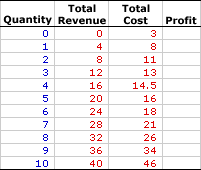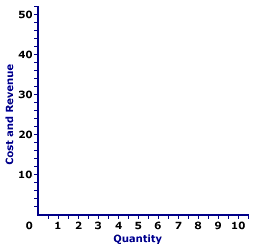Thursday  September 23, 2021
 AmosWEB means Economics with a Touch of Whimsy!MARGINAL PROPENSITY TO IMPORT: The proportion of each additional dollar of household income that is used for imports. Or alternatively, this is the change in imports due to a change in disposable income. Abbreviated MPI, the marginal propensity to import plays a minor role in modifying the aggregate expenditure line and the multiplier effect.PERFECT COMPETITION, TOTAL ANALYSIS:

A perfectly competitive firm produces the profit-maximizing quantity of output that generates the greatest difference between total revenue and total cost. This total approach is one of three methods that used to determine the profit-maximizing quantity of output. The other two methods involve the direct analysis of economic profit or a comparison of marginal revenue and marginal cost.
Perfect competition is a market structure characterized by a large number of small firms producing identical products with perfect resource mobility and perfect knowledge. These conditions mean a perfectly competitive firm faces a horizontal, or perfectly elastic, demand curve. With this horizontal demand curve, marginal revenue is equal to average revenue, both of which are also equal to price.

Comparable to any profit-maximizing firm, a perfectly competitive firm produces the quantity of output in the short run that generates the maximum difference between total revenue with total cost, which is economic profit. At this production level, the firm cannot increase profit by changing the level of production. The analysis of total revenue and total cost can be achieved through a table of numbers or with total revenue and total cost curves.

### Working the Numbers

A perfectly competitive firm is presumed to produce the quantity of output that maximizes economic profit--the difference between total revenue and total cost. This decision can be analyzed using the exhibit below. This table presents revenue and cost information for Phil the zucchini grower, a hypothetical example of a perfectly competitive firm.

Because the zucchinis that Phil produces are identical to those produced by gadzillions of other zucchini growers, he has no market control and must sell his zucchinis at the going market price of \$4 per pound. Phil is a price taker. Phil's status as a perfectly competitive, price-taking firm is reflected in this table.

The Zucchini Numbers• Quantity: The first column presents the quantity of output produced, ranging from 0 to 10 pounds of zucchinis.

• Total Revenue: The second column presents total revenue, ranging from a low of \$0 for no output to a high or \$40 for 10 pounds of zucchinis. If Phil sells 1 pound of zucchinis, he receives \$4 in total revenue. Total revenue is \$8 for 2 pounds of zucchinis. The total revenue in the second column is the \$4 market price times the quantity of zucchinis sold.

• Total Cost: The third column presents the total cost incurred by the firm in the production of this output, ranging from a low of \$3 for zero output to a high of \$46 for 10 pounds of zucchinis. Because Phil has fixed cost, he incurs \$3 of total cost even though he produces no zucchinis. Producing 1 pound of zucchinis incurs a total cost of \$8. Producing 2 pounds of zucchinis incurs a total cost of \$11. Total cost rises as Phil produces more.

• Profit: The fourth column at the far right of the table is available to display economic profit, the difference between total revenue in the second column and total cost in the third column. A click of the [Profit] button displays Phil's economic profit in the fourth column.
The task is to determine the economic profit Phil earns for each quantity of zucchinis produced, then to identify which zucchini production level provides the maximum profit.

A click of the [Incurring Losses] button indicates that producing and selling 1 pound of zucchini generates an economic loss of \$4. Total revenue is \$4 and total cost is \$8. A \$3 loss results from 2 pounds of zucchinis. In fact, Phil incurs an economic loss for the first 3 pounds of zucchinis. Phil also incur an economic lose by producing 10 pounds of zucchinis.

But loss is not what Phil seeks. Click the [Earning Profits] button to highlight the range of production levels that generate positive economic profit. Phil initially turns his profit picture around with 4 pounds of zucchinis. At 4 pounds of zucchinis, Phil's total revenue is less than his total cost by \$1.50. Profit remains positive through the production of 9 pounds of zucchinis, Phil's total revenue exceeds his total cost and Phil receives an economic profit. For 6 pounds of zucchinis, this profit is \$6, for 7 profit is \$7, and for 8 profit drops back to \$6 again.

So what is the profit-maximizing level of zucchini production Phil should undertake? The desired production level is clearly not 3 pounds or less, nor is it 10 pounds (or more), all of which lead to economic loss. It must be within the highlighted range between 4 and 9 pounds of zucchinis.

The quantity that generates the greatest of economic profit is 7 pounds of zucchinis. This alternative can be highlighted by clicking the [Profit Max] button. The production of 7 pounds of zucchinis results in \$28 of total revenue and \$21 of total cost, a difference of \$7. No other production level generates a greater economic profit. Producing 1 more pound of zucchinis or 1 less pound of zucchinis reduces profit to \$6.

### Working the Curves

The Zucchini CurvesThe short-run production decision for a perfectly competitive firm can be graphically illustrated using total revenue and total cost curves. The exhibit to the right is standing poised to display these curves.
• Total Revenue: A click of the [Total Revenue] button reveals a straight green line labeled TR that depicts the total revenue Phil receives from zucchini production. The line is straight, with a constant slope, because the price is constant at \$4.

• Total Cost: A click of the [Total Cost] button reveals a curvy red line labeled TC represents the total cost Phil incurs in the production of zucchinis. The shape of this curve is based on increasing, then decreasing marginal returns.

• Profit: The vertical difference between these two lines is economic profit. If the total revenue line is above the total cost line in the middle of the diagram, economic profit is positive. If the total revenue line is below the total cost line at the far right and far left, economic profit is negative.
The key for Phil is to identify the production level that gives the greatest vertical distance between the total revenue and total cost curves in the middle of the diagram. This might not be evident by just looking at the exhibit, but it can be illustrated by clicking the [Profit Max] button. The output quantity identified is, once again, 7 pounds of zucchinis.

Before leaving this graph, two other quantities can be highlighted. The total revenue and total cost curves intersect at two quantities--at about 3.5 pounds of zucchinis and just over 9 pounds of zucchinis. Click the [Breakeven] button to highlight these two output levels.

Both quantities are termed breakeven output. Breakeven output is a quantity of output in which the total revenue is equal to total cost such that a firm earns exactly a normal profit, and thus receives no economic profit nor incurs an economic loss. The reason for the term "breakeven" output is that the firm is just "breaking even." It is neither making a profit nor incurring a loss. Economic profit is zero. Breakeven output is usually most noteworthy as a reference point. The profit-maximizing production level invariably occurs between the two breakeven output levels.

 <= PERFECT COMPETITION, SHUTDOWN PERFECTLY ELASTIC =>Recommended Citation:

PERFECT COMPETITION, TOTAL ANALYSIS, AmosWEB Encyclonomic WEB*pedia, http://www.AmosWEB.com, AmosWEB LLC, 2000-2021. [Accessed: September 23, 2021].

Check Out These Related Terms...

Or For A Little Background...

And For Further Study...
Search Again?PURPLE SMARPHIN[What's This?] Today, you are likely to spend a great deal of time watching the shopping channel looking to buy either a computer that can play video games and burn DVDs or a black duffle bag with velcro closures. Be on the lookout for deranged pelicans.Your Complete ScopeJunk bonds are so called because they have a better than 50% chance of default, carrying a Standard & Poor's rating of CC or lower."People of mediocre ability sometimes achieve outstanding success because they don't know when to quit. "-- George Allen, U.S. senatorLPGLiquid Petroleum GasA PEDestrian's Guide Xtra CreditTell us what you think about AmosWEB. Like what you see? Have suggestions for improvements? Let us know. Click the User Feedback link.| | | | | | | | | | |
| | | |

Thanks for visiting AmosWEB